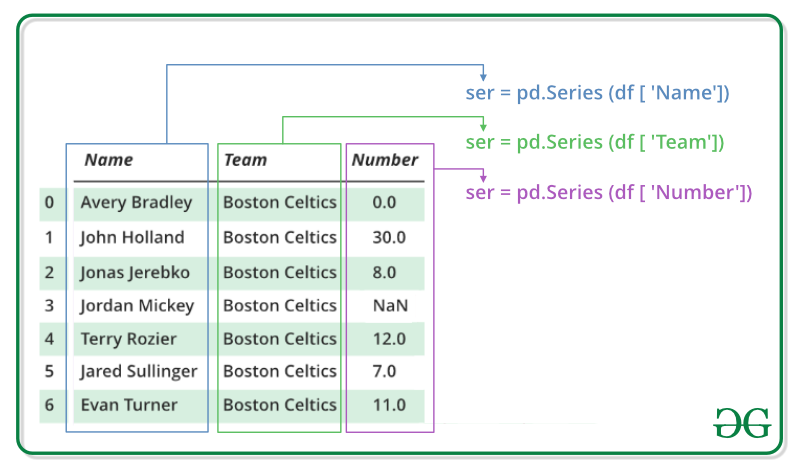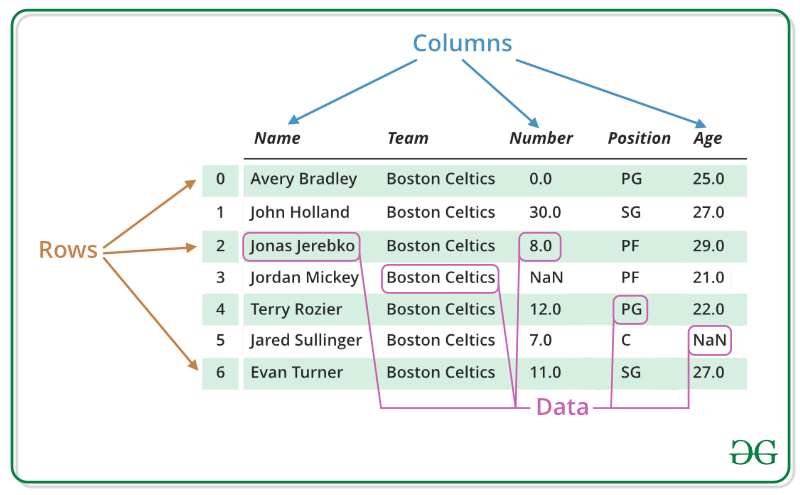# Introduction to Pandas in Python

Pandas is an open-source library that is made mainly for working with relational or labeled data both easily and intuitively. It provides various data structures and operations for manipulating numerical data and time series. This library is built on the top of the NumPy library. Pandas is fast and it has high-performance & productivity for users.

Table of Content

## History

Pandas was initially developed by Wes McKinney in 2008 while he was working at AQR Capital Management. He convinced the AQR to allow him to open source the Pandas. Another AQR employee, Chang She, joined as the second major contributor to the library in 2012. Over the time many versions of pandas have been released. The latest version of the pandas is 1.0.1

• Fast and efficient for manipulating and analyzing data.
• Data from different file objects can be loaded.
• Easy handling of missing data (represented as NaN) in floating point as well as non-floating point data
• Size mutability: columns can be inserted and deleted from DataFrame and higher dimensional objects
• Data set merging and joining.
• Flexible reshaping and pivoting of data sets
• Provides time-series functionality.
• Powerful group by functionality for performing split-apply-combine operations on data sets.

## Getting Started

After the pandas has been installed into the system, you need to import the library. This module is generally imported as –

`import pandas as pd`

Here, pd is referred to as an alias to the Pandas. However, it is not necessary to import the library using alias, it just helps in writing less amount of code everytime a method or property is called.

Pandas generally provide two data structure for manipulating data, They are:

• Series
• DataFrame

### Series

Pandas Series is a one-dimensional labeled array capable of holding data of any type (integer, string, float, python objects, etc.). The axis labels are collectively called index. Pandas Series is nothing but a column in an excel sheet. Labels need not be unique but must be a hashable type. The object supports both integer and label-based indexing and provides a host of methods for performing operations involving the index.#### Creating a Series

In the real world, a Pandas Series will be created by loading the datasets from existing storage, storage can be SQL Database, CSV file, and Excel file. Pandas Series can be created from the lists, dictionary, and from a scalar value etc.

Example:

 `import` `pandas as pd  ` `import` `numpy as np ` ` `  ` `  `# Creating empty series  ` `ser ``=` `pd.Series()  ` `   `  `print``(ser)  ` ` `  `# simple array  ` `data ``=` `np.array([``'g'``, ``'e'``, ``'e'``, ``'k'``, ``'s'``])  ` `   `  `ser ``=` `pd.Series(data)  ` `print``(ser) `

Output:

```Series([], dtype: float64)
0    g
1    e
2    e
3    k
4    s
dtype: object
```

### DataFrame

Pandas DataFrame is two-dimensional size-mutable, potentially heterogeneous tabular data structure with labeled axes (rows and columns). A Data frame is a two-dimensional data structure, i.e., data is aligned in a tabular fashion in rows and columns. Pandas DataFrame consists of three principal components, the data, rows, and columns.#### Creating a DataFrame

In the real world, a Pandas DataFrame will be created by loading the datasets from existing storage, storage can be SQL Database, CSV file, and Excel file. Pandas DataFrame can be created from the lists, dictionary, and from a list of dictionary etc.

Example:

 `import` `pandas as pd  ` `   `  `# Calling DataFrame constructor  ` `df ``=` `pd.DataFrame()  ` `print``(df) ` ` `  `# list of strings  ` `lst ``=` `[``'Geeks'``, ``'For'``, ``'Geeks'``, ``'is'``,   ` `            ``'portal'``, ``'for'``, ``'Geeks'``]  ` `   `  `# Calling DataFrame constructor on list  ` `df ``=` `pd.DataFrame(lst)  ` `print``(df)  `

Output:

```Empty DataFrame
Columns: []
Index: []
0
0   Geeks
1     For
2   Geeks
3      is
4  portal
5     for
6   Geeks```

## Why Pandas is used for Data Science

Pandas is generally used for data science but have you wondered why? This is because pandas is used in conjunction with other libraries that are used for data science. It is built on the top of the NumPy library which means that a lot of structures of NumPy are used or replicated in Pandas. The data produced by Pandas is often used as input for plotting functions of Matplotlib, statistical analysis in SciPy, machine learning algorithm in Scikit-learn.

Pandas program can be run from any text editor but it is recommended to use Jupyter Notebook for this as Jupyter given the ability to execute code in a particular cell rather than executing the entire file. Jupyter also provides an easy way to visualize pandas dataframe and plots.

Note: For more information on Jupyter Notebook, refer to How To Use Jupyter Notebook – An Ultimate Guide

My Personal Notes arrow_drop_upCheck out this Author's contributed articles.

If you like GeeksforGeeks and would like to contribute, you can also write an article using contribute.geeksforgeeks.org or mail your article to contribute@geeksforgeeks.org. See your article appearing on the GeeksforGeeks main page and help other Geeks.

Please Improve this article if you find anything incorrect by clicking on the "Improve Article" button below.

Article Tags :

3

Please write to us at contribute@geeksforgeeks.org to report any issue with the above content.